Courses

# Normal Subgroups,Quotient Groups and Homomorphisms Mathematics Notes | EduRev

## Mathematics : Normal Subgroups,Quotient Groups and Homomorphisms Mathematics Notes | EduRev

The document Normal Subgroups,Quotient Groups and Homomorphisms Mathematics Notes | EduRev is a part of the Mathematics Course Algebra for IIT JAM Mathematics.
All you need of Mathematics at this link: Mathematics

A normal subgroup is a subgroup that is invariant under conjugation by any element of the original group: H is normal if and only if gHg-1 = H for any g ∈ G. Equivalently, a subgroup H of G is normal if and only if gH = Hg for any g ∈ G.

Normal subgroups are useful in constructing quotient groups, and in analyzing homomorphisms.

Quotient Groups

A quotient group is defined as G/N for some normal subgroup N of G, which is the set of cosets of N w.r.t. G, equipped with the operation º satisfying for all g, h ∈ G.

This definition is the reason that N must be normal to define a quotient group; it holds because the chain of equalities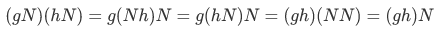holds, where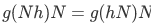utilizes the fact that Nh = hN for any h (true iff N is normal, by definition).

For example, consider the subgroup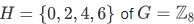(which is an additive group). The left cosets are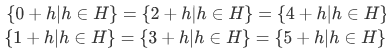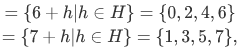so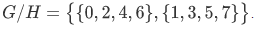This can be more cleanly written as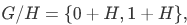which is isomorphic to {0,1} or the cyclic group C2. Additional examples:

• The quotient group Z/2Z, where 2Z--t the group of even integers--is a normal subgroup of , is isomorphic to  as well.
• The quotient group R/Z, where Z--the group of integers--is a normal subgroup of the reals , is isomorphic to the circle group defined by the complex numbers with magnitude 1.

Homomorphisms and Normal Subgroups

Recall that a homomorphism from G to H is a function φ such that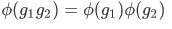for all g1, g2 ∈ G.

The kernel of a homomorphism is the set of elements of G that are sent to the identity in H, and the kernel of any homomorphism is necessarily a normal subgroup of G.

In fact, more is true: the image of G under this homomorphism (the set of elements G is sent to under φ) is isomorphic to the quotient group G/ker(φ), by the first isomorphism theorem. This provides a bijection between normal subgroups of G and the set of images of G under homomorphisms.

Thus normal subgroups can be classified in another manner:

 A subgroup N of G is normal if and only if there exists a homomorphism on G whose kernel is N.
Offer running on EduRev: Apply code STAYHOME200 to get INR 200 off on our premium plan EduRev Infinity!

58 docs

,

,

,

,

,

,

,

,

,

,

,

,

,

,

,

,

,

,

,

,

,

,

,

,

;